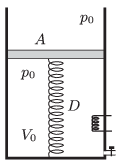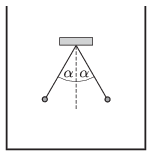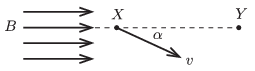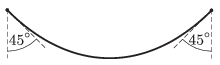Mathematical and Physical Journal
for High Schools
Issued by the MATFUND Foundation
 Already signed up? New to KöMaL?

# KöMaL Problems in Physics, May 2015

Show/hide problems of signs:## Problems with sign 'M'

Deadline expired on June 10, 2015.

M. 351. Measure the equivalent series resistance of an electrolytic capacitor. How does it depend on the applied voltage?

(6 pont)

solution, statistics## Problems with sign 'P'

Deadline expired on June 10, 2015.

P. 4736. A ship travels along the river from one bridge to another, and when it reaches the second bridge it turns back immediately. By what factor is the time of the round trip on the river greater than that of in still water?

(3 pont)

solution, statistics

P. 4737. A light ray is incident on one of the faces of a glass prism perpendicularly to it. The angle of refraction of the light ray on another face of the prism is $\displaystyle 45^\circ$. What is the apex angle of the prism if the speed of light in this glass prism is 240000 km/s?

(3 pont)

solution, statistics

P. 4738. At what minimum speed should we have to go next to a picket fence, in order to sense the light, passing the fence perpendicularly, continuous? The width of the pickets is 5 cm and the width of the gaps between them is 10 cm. The human eye cannot distinguish between images which lasts less than one-fifteenth of a second.

(3 pont)

solution, statistics

P. 4739. A red and a green stone fall freely next to each other along vertical paths at a place of the universe where the acceleration due to gravity is exactly 10 m/s$\displaystyle {}^2$ and where nothing prevents free fall. The red stone just starts falling when the green stone reaches it. The green stone also started from rest but from a higher position. After a while the distance between the two stones is 7 m, and then after two more seconds elapses the distance between them will increase to 27 m. How much higher did the green stone start?

(4 pont)

solution, statistics

P. 4740. There is a sample of ideal gas in a vertical cylinder, which is closed at its lower base. At the top there is a massless frictionlessly moveable piston. The volume of the sample of ideal gas is $\displaystyle V_0=8~\rm dm^3$, and its pressure is the same as the ambient air pressure, which is $\displaystyle p_0=10^5$ Pa. The piston is attached to the bottom of the cylinder with a spring of spring constant $\displaystyle D=1000$ N/m. The area of the bottom of the cylinder is $\displaystyle A=2~\rm dm^2$. During a physics experiment the mass of the gas is increased by 50% through a tap on the cylinder, and by heating the absolute temperature of the gas is increased by 60%. Determine the volume and the pressure of the gas after these changes.(4 pont)

solution, statistics

P. 4741. A 20-litre gas cylinder just runs out'' of gas at the foot of a high hill next to the seashore. Then immediately the valve is closed, and the gas cylinder is carried up to the research laboratory at the top of the hill. Here some gas from the cylinder can again be used. When finally the gas cylinder is exhausted again its mass is measured, and it turns out that due to using the gas at the top of the hill its mass decreased by 4.1 grams. The air pressure at the top of the hill is 32 kPa less than at sea level. Both at the sea level and at the laboratory the temperature of the gas is $\displaystyle 27~{}^\circ$C, and the density of air at sea level is 1.2 kg/m$\displaystyle {}^3$.

$\displaystyle a)$ What type of gas was in the cylinder?

$\displaystyle b)$ How high is the hill?

(4 pont)

solution, statistics

P. 4742. A capacitor of capacitance $\displaystyle C$ is charged, and it stores $\displaystyle W$ electrical energy. Then another neutral capacitor is connected to it in parallel. What should the capacitance of the second condenser be in order that after the equilibrium is gained the energy of the second condenser is to be the greatest?

What will the total energy stored in the two condensers be in this case?

(4 pont)

solution, statistics

P. 4743. Two insulating threads, which have the same lengths, are suspended at the same point and at their lower end of each, there is a small ebony ball attached. The balls are given the same amount of like charges. The angle between the threads is $\displaystyle 2\alpha=60^\circ$ when the pendulums are inside a container at rest in air. Then the container is filled with petroleum, such that both balls are in the petroleum, far from the walls of the container and from the surface of the liquid. What is the angle between the threads now?Data: The density of ebony is $\displaystyle 1200~{\rm kg}/{\rm m^3}$, the density of petroleum is $\displaystyle 820~{\rm kg}/{\rm m^3}$. The relative dielectric constant of petroleum is $\displaystyle \varepsilon_{\rm r}=2$.

(5 pont)

solution, statistics

P. 4744. There are two points ($\displaystyle X$ and $\displaystyle Y$) on the same $\displaystyle B$-line at a distance of 10 cm in a uniform magnetic field of magnetic induction $\displaystyle B=0.02$ tesla. An electron, which was accelerated through a potential difference of 800 V, passes point $\displaystyle X$, such that its velocity encloses an angle of $\displaystyle \alpha$ with the induction line. What may the value of $\displaystyle \alpha$ be if the electron passes point $\displaystyle Y$ as well?(5 pont)

solution, statistics

P. 4745. The topic of a physics lesson is the decibel scale. One of the students asks the teacher whether there is an upper limit of the maximum intensity sound. The answer is yes, the theoretical upper limit for the intensity of sound is 194 dB''. How is this strange value gained?

(5 pont)

solution, statistics

P. 4746. The following experiment is usually shown in order to demonstrate that light is a transverse wave:

A narrow parallel beam of natural light hits a plane glass, such that the reflected and the refracted rays are perpendicular to each other. Then another plane glass is used to reflect the reflected ray, such that the angle of incidence is the same as in the previous case. If this latter glass is rotated about an axis which coincides with the incident light ray, then it may happen that there is no reflected ray from this piece of glass.

$\displaystyle a)$ How does this experiment proves that light is transverse wave?

$\displaystyle b)$ What is the angle of incidence, if the refractive index of the glass is $\displaystyle n=1.5$?

$\displaystyle c)$ What is the angle between the planes of the two pieces of glass when there is no reflected ray from the second glass?

(5 pont)

solution, statistics

P. 4747. The two endpoints of a 40 cm long chain are fixed at the same height as shown in the figure. What is the radius of the curvature of the chain at$\displaystyle a)$ its lowest point;

$\displaystyle b)$ the points where the chain is suspended?

(6 pont)

solution, statistics